You may also likeChocolate

There are three tables in a room with blocks of chocolate on each. Where would be the best place for each child in the class to sit if they came in one at a time?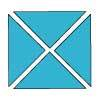Four Triangles Puzzle

Cut four triangles from a square as shown in the picture. How many different shapes can you make by fitting the four triangles back together?Cut it Out

Can you dissect an equilateral triangle into 6 smaller ones? What number of smaller equilateral triangles is it NOT possible to dissect a larger equilateral triangle into?

Table Patterns Go Wild!

Age 7 to 11Challenge Level

Nearly all of us have made table patterns on hundred squares, that is $10$ by $10$ grids. Some tables made vertical lines, some made diagonal lines and some patterns ranging across the whole space. Hundred squares are $10$ by $10$ grids. In this problem we will call these "$10$ grids".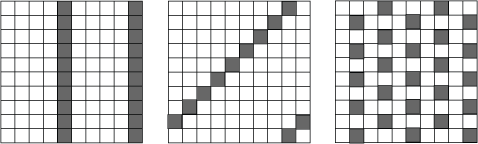What numbers made which sort of patterns and why?

This problem looks at the patterns on differently sized square grids. These are from $4$ grids (that is a $4$ by $4$ grid) to $9$ grids.

These are patterns on a $7$, a $5$, an $8$ and on a $6$ grid: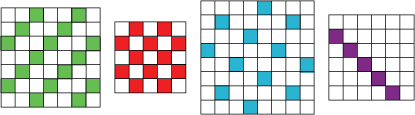What tables made these patterns? Can you think why they made them like that?

Perhaps this is the time to experiment for yourself. You can use grids drawn on squared paper or use this sheet.
Can you discover what makes vertical and diagonal lines on the different grids and what makes the various patterns. Can you make the checked pattern? What table do you need to use on what kind of grid?

Here are the top parts of some grids.
Can you identify what table on what grid have been used to make them?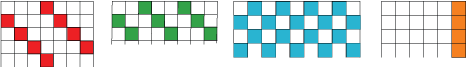Here are some parts of various grids. This time we have not shown the edges of the grids. Can you identify what tables on what grids could have been used to make these patterns?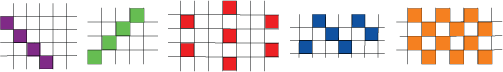There may be more than one answer.